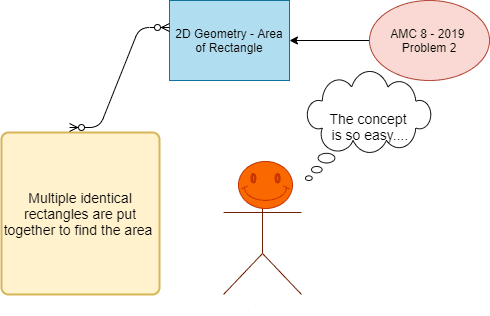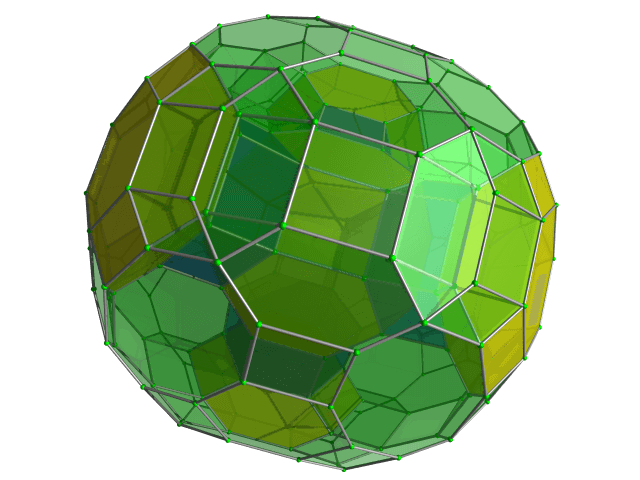Categories

# 2D Geometry AMC 8, 2019 Problem 2

Try this beautiful problem from AMC 10. It involves geometry of triangles. We provide sequential hints so that you can try the problem.

## Competency in Focus: 2D Geometry (Area of Rectangle)

This problem from American Mathematics contest (AMC 8, 2019) is based on calculation of area of  Rectangle .

## First look at the knowledge graph## Next understand the problem

Three identical rectangles are put together to form rectangle $ABCD$, as shown in the figure below. Given that the length of the shorter side of each of the smaller rectangles is 5 feet, what is the area in square feet of rectangle $ABCD$?##### Source of the problem
American Mathematical Contest 2019, AMC 8  Problem 2

### 2D Geometry (Area of Rectangle)

3/10
##### Suggested Book
Challenges and Thrills in Pre College Mathematics

Do you really need a hint? Try it first!
The length of the smaller side of each rectangle is given 5 feet. So using the diagram we can find  that the larger side of the small rectangle is 2 times the length of the smaller side.
So find the longer side which is 5*2 = 10 feet.
the area of the identical rectangles is 5*10 = 50 square feet
Thus at end we have 3 identical rectangles formed a bigger rectangle……Try to solve …   50*3 = 150 square feet

## AMC - AIME Program

AMC - AIME - USAMO Boot Camp for brilliant students. Use our exclusive one-on-one plus group class system to prepare for Math Olympiad## External Tangent | AMC 10A, 2018 | Problem 15

Try this beautiful Problem on geometry based on circle from AMC 10A, 2018. Problem-15. You may use sequential hints to solve the problem.

## Dice Problem | AMC 10A, 2014| Problem No 17

Try this beautiful Problem on Probability from AMC 10A, 2014. Problem-17, You may use sequential hints to solve the problem.

## Problem on Curve | AMC 10A, 2018 | Problem 21

Try this beautiful Problem on Co-ordinate geometry from AMC 10A, 2018. Problem-21, You may use sequential hints to solve the problem.

## Right-angled Triangle | AMC 10A, 2018 | Problem No 16

Try this beautiful Problem on triangle from AMC 10A, 2018. Problem-16. You may use sequential hints to solve the problem.

## Finding Greatest Integer | AMC 10A, 2018 | Problem No 14

Try this beautiful Problem on Algebra from AMC 10A, 2018. Problem-14, You may use sequential hints to solve the problem.

## Length of the crease | AMC 10A, 2018 | Problem No 13

Try this beautiful Problem on triangle from AMC 10A, 2018. Problem-13. You may use sequential hints to solve the problem.

## Right-angled shaped field | AMC 10A, 2018 | Problem No 23

Try this beautiful Problem on triangle from AMC 10A, 2018. Problem-23. You may use sequential hints to solve the problem.

## Area of region | AMC 10B, 2016| Problem No 21

Try this beautiful Problem on Geometry on Circle from AMC 10B, 2016. Problem-20. You may use sequential hints to solve the problem.

## Coin Toss Problem | AMC 10A, 2017| Problem No 18

Try this beautiful Problem on Probability from AMC 10A, 2017. Problem-18, You may use sequential hints to solve the problem.

## GCF & Rectangle | AMC 10A, 2016| Problem No 19

Try this beautiful Problem on Geometry on Rectangle from AMC 10A, 2010. Problem-19. You may use sequential hints to solve the problem.

This site uses Akismet to reduce spam. Learn how your comment data is processed.